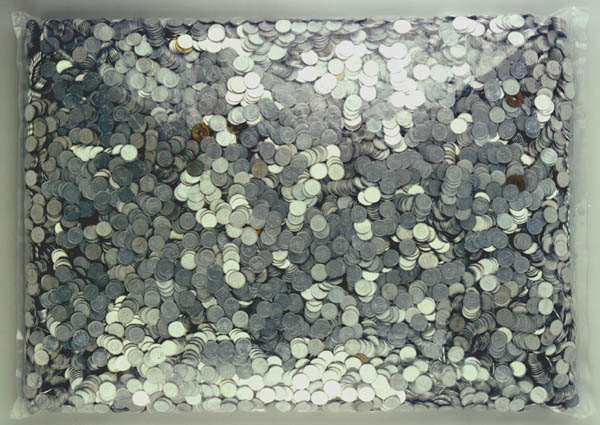to english | index | next

17296枚の硬貨から成る18416円 (金額第一五番)
18416 Yen Which Consists of 17296 Coins (Money Amount No. 15)

Japanese coins, a plastic bag
2001
photo by Takagi Shigeki

cf. 17296 and 18416 are the third smallest amicable pair which Fermat found in 1636.
(Two numbers are called amicable if each equals to the sum of the divisors of the other.)
Divisors of 17296: 1 + 2 + 4 + 8 + 16 + 23 + 46 + 47 + 92 + 94 + 184 + 188 + 368 + 376 + 752 + 1081 + 2162 + 4324 + 8648 = 18416
Divisors of 18416: 1 + 2 + 4 + 8 + 16 + 1151 + 2302 + 4604 + 9208 = 17296